## Prime Factorization and the Least Common Multiple

### Learning Outcomes

• Find the prime factorization of a number
• Find the least common multiple of a list of numbers

The word factor can be both a noun and a verb. To factor a number is to rewrite it by breaking it up into a product of smaller numbers. For example, we can factor 24 by writing it as $6\ast4$. We say that 6 and 4 are factors of 24. But so are 2 and 12. There are many ways to write this number.

A useful skill to have when doing algebra is the ability to rewrite numbers and expressions in helpful forms.  For example, consider some different forms of the number 24.

$24 \qquad \dfrac{72}{3} \qquad \sqrt{576} \qquad 6\ast4 \qquad 2\cdot2\cdot2\cdot3$.

Composite numbers, like 24, are natural numbers that can be written as products of other natural numbers. Prime numbers are natural numbers that have only two possible factors, themselves and the number 1. The final form of 24 in the list above, $2\cdot2\cdot2\cdot3$, is called its prime factorization. When we write the prime factorization of a number, we are writing it as a product of only its prime factors. Being able to find the prime factorization of a composite number is an especially useful skill to have when doing algebra.

### Prime Factorization

The prime factorization of a number is the product of prime numbers that equals the number.

You may want to refer to the following list of prime numbers less than $50$ as you work through this section.

$2,3,5,7,11,13,17,19,23,29,31,37,41,43,47$

Tip: Knowing the first five prime numbers will come in handy when reducing fractions.

### Prime Factorization Using the Factor Tree Method

One way to find the prime factorization of a number is to make a factor tree. We start by writing the number, and then writing it as the product of two factors. We write the factors below the number and connect them to the number with a small line segment—a “branch” of the factor tree.

If a factor is prime, we circle it (like a bud on a tree), and do not factor that “branch” any further. If a factor is not prime, we repeat this process, writing it as the product of two factors and adding new branches to the tree.

We continue until all the branches end with a prime. When the factor tree is complete, the circled primes give us the prime factorization.

For example, let’s find the prime factorization of $36$. We can start with any factor pair such as $3$ and $12$. We write $3$ and $12$ below $36$ with branches connecting them.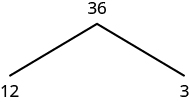The factor $3$ is prime, so we circle it. The factor $12$ is composite, so we need to find its factors. Let’s use $3$ and $4$. We write these factors on the tree under the $12$.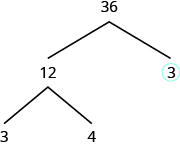The factor $3$ is prime, so we circle it. The factor $4$ is composite, and it factors into $2\cdot 2$. We write these factors under the $4$. Since $2$ is prime, we circle both $2\text{s}$.The prime factorization is the product of the circled primes. We generally write the prime factorization in order from least to greatest.

$2\cdot 2\cdot 3\cdot 3$

In cases like this, where some of the prime factors are repeated, we can write prime factorization in exponential form.

$\begin{array}{c}2\cdot 2\cdot 3\cdot 3\\ \\ {2}^{2}\cdot {3}^{2}\end{array}$

Note that we could have started our factor tree with any factor pair of $36$. We chose $12$ and $3$, but the result would have been the same if we had started with $2$ and $18, 4$ and $9,\text{ or }6\text{ and }6$.

### Find the prime factorization of a composite number using the tree method

1. Find any factor pair of the given number, and use these numbers to create two branches.
2. If a factor is prime, that branch is complete. Circle the prime.
3. If a factor is not prime, write it as the product of a factor pair and continue the process.
4. Write the composite number as the product of all the circled primes.

### example

Find the prime factorization of $48$ using the factor tree method.

Solution:

 We can start our tree using any factor pair of $48$. Let’s use $2\text{ and }24$. We circle the $2$ because it is prime and so that branch is complete.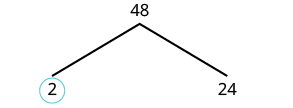Now we will factor $24$. Let’s use $4\text{ and }6$.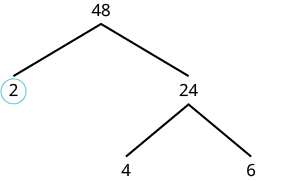Neither factor is prime, so we do not circle either.We factor the $4$, using $2\text{ and }2$.We factor $6\text{, using }2\text{ and }3$. We circle the $2\text{s and the }3$ since they are prime. Now all of the branches end in a prime.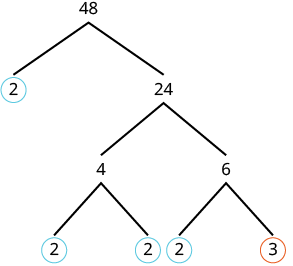Write the product of the circled numbers. $2\cdot 2\cdot 2\cdot 2\cdot 3$ Write in exponential form. ${2}^{4}\cdot 3$

Check this on your own by multiplying all the factors together. The result should be $48$.

### try it

The following video shows how to find the prime factorization of $60$ using the factor tree method.

### example

Find the prime factorization of $84$ using the factor tree method.

### try it

There are other methods that work well to find the prime factorization of a number. Any method that factors out small primes repeatedly until there are only prime factors remaining is acceptable. See the video below for a demonstration of using stacked division to find a prime factorization.

### Finding Least Common Multiples

Consider the multiples of two numbers, 3 and 4. What is the least common multiple between them?

Certainly 3 and 4 have many multiples in common: 12, 24, 36, etc. But 12 is the smallest among them.

The smallest number that is a multiple of two numbers is called the least common multiple (LCM). So the LCM of $3$ and $4$ is $12$.

One of the reasons we find prime factorizations is to use them to find the least common multiple of two or more numbers. This will be useful when we add and subtract fractions with different denominators.

### Prime Factors Method

We can find the least common multiple of two numbers by inspecting their prime factors. We’ll use this method to find the LCM of $12$ and $18$.

We start by finding the prime factorization of each number.

$12=2\cdot 2\cdot 3 \qquad$  and $\qquad 18=2\cdot 3\cdot 3$

Then we find the largest instance of each prime appearing in any one factorization. Here, we see that the number 2 appears twice in the factorization of 12, but only once in 18. And the number 3 appears twice in 18, but only once in 12. So, we select $2\cdot2$ and  $3\cdot3$ and multiply them together to find the LCM.

$12=2\cdot 2\cdot 3 \qquad \text{ and } \qquad 18=2\cdot 3\cdot 3$

$\qquad 2\cdot2 \qquad \qquad \ast \qquad \qquad 3\cdot3$.

$\qquad\quad \qquad 2\cdot2\cdot3\cdot3=36$.

The LCM of 12 and 18 is 36.

If a prime appears the same number of times in any factorization, just select one instance. Let’s find the LCM of a list of numbers as an example.

Find the LCM of 12, 18, 20, and 60. First we’ll write the numbers as products of primes.

$12 = 2\cdot2\cdot3 \qquad 18=2\cdot3\cdot3 \qquad 20=2\cdot2\cdot5 \qquad 60=2\cdot2\cdot3\cdot5$

We see that the largest instance of the number 2 appears in the numbers 12, 20, and 60. But we just select one largest instance. The largest instance of the number 3 appears in 18, where there are two factors of 3. And the number 5 appears once in 20 and once in 60, so we’ll select one 5.

The LCM of 12, 18, 20, and 60 is

$2\cdot2\cdot3\cdot3\cdot5 = 180$.

So, 180 is the smallest number that 12, 18, 20, and 60 all divide evenly into.

### Find the LCM using the prime factors method

1. Find the prime factorization of each number.
2. Inspect each factorization for the largest number of instances of each prime number appearing in each factorization
3. Select one set of each largest instance of each prime factor appearing.
4. Multiply the selected factors together to obtain the LCM.

It can be helpful to write the prime factors next to each number, matching them vertically in columns, then bringing down the primes in each column to collect the factors whose product is the LCM. The following examples illustrate this technique.

### example

Find the LCM of $15$ and $18$ using the prime factors method.

### example

Find the LCM of $50$ and $100$ using the prime factors method.

### try it

In the next video we see how to find the Least Common Multiple by using prime factorization.